# RS Aggarwal Class 7 Solutions Chapter 10 Percentage Ex 10B

In this chapter, we provide RS Aggarwal Solutions for Class 7 Chapter 10 Percentage Ex 10B for English medium students, Which will very helpful for every student in their exams. Students can download the latest RS Aggarwal Solutions for Class 7 Chapter 10 Percentage Ex 10B Maths pdf, free RS Aggarwal Solutions Class 7 Chapter 10 Percentage Ex 10B Maths book pdf download. Now you will get step by step solution to each question.

### RS Aggarwal Solutions for Class 7 Chapter 10 Percentage Ex 10B Download PDF

Question 1.
Solution:
Rupesh seemed 495 marks out of 750
Percentage of marks = 495750 x 100 = 66%

Question 2.
Solution:
Monthly salary = Rs. 15625
Increase = 12%
Amount of increase = 15625×12100 = Rs. 1875
New salary = Rs. 15625 + Rs. 1875 = Rs. 17500

Question 3.
Solution:
Excise duty in the beginning = Rs. 950
Reduced duty = Rs. 760
Reduction = Rs. 950 – Rs. 760 = Rs. 190
Reduction percent = 190×100950 = 20%

Question 4.
Solution:
Let x be the total cost of the T.V
96% of x = 10464Total cost of T.V = Rs. 10900

Question 5.
Solution:
Let number of students = x
In a school boys = 70%
girls = 100 – 70 = 30%
Now 30% of x = 504Number of boys = 1680 – 504 = 1176
and number of total students = 1680

Question 6.
Solution:
Copper required = 69 kg
copper in ore = 12%
Let quantity of ore = x kg
12% of x = 69Quantity of ore = 575kg

Question 7.
Solution:
Pass marks = 36%
A students gets marks = 123
But failed by 39 marks
Pass marks = 123 + 39 = 162
Now, 36% of maximum marks = 162
Maximum marks = 162×10036 = 450 marks

Question 8.
Solution:
Let number of apples = x
Number of apples sold = 40% of x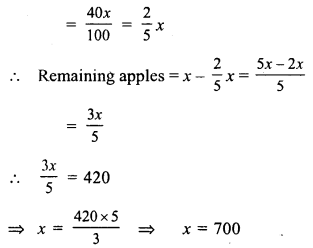Hence number of apples = 700

Question 9.
Solution:
Let total number of examinees = x
the numbers of examinees who passed = 72% of x
= xx72100
= 18×25Question 10.
Solution:
Let the gross value of moped = x
Amount of commission = 5% of xQuestion 11.
Solution:
Total gunpowder = 8 kg
Amount of nitre = 75%
amount of sulphur = 10%
Rest of powder which is charcoal = 100 – (75 + 10) = 100 – 85 = 15 = 15%
Amount of charcoal = 8 x 15100 = 120100
= 65 kg = 1 kg 200 grams = 1.2 kg

Question 12.
Solution:
Quantity of chalk = 1 kg or 1000 gQuestion 13.
Solution:
Let total number of days on which the
school open = x
and Sonal’s attendance = 75%
x x 75% = 219No. of days on which was school open = 292 days

Question 14.
Solution:
Rate of commission = 3%
Amount of commission = Rs. 42660
Let value of property = x
then 3% of x = 42660Question 15.
Solution:
Total votes of the constituency = 60000
= 80100 x 60000 = 48000
= 60100 x 48000 = 28800
Votes polled in favour of B = 48000 – 28800 = 19200

Question 16.
Solution:
Let original price of shirt = Rs. x
Discount = 12%Original price of shirt = Rs. 1350

Question 17.
Solution:
Let original price of sweater = x
Rate of increase = 8%
Increased price = x + 8% of x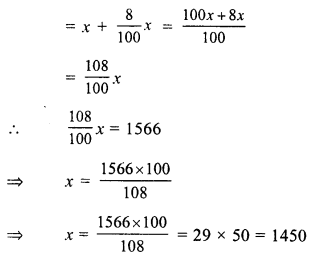Hence, original price of sweater = Rs. 1450

Question 18.
Solution:
Let total income = x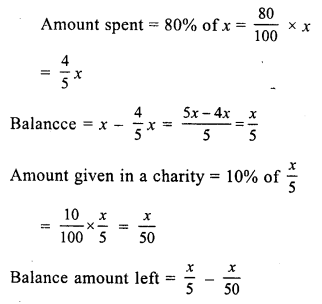Question 19.
Solution:
Let the given number = 100
Then increase % = 20%Decrease = 100 – 96 = 4
Decrease per cent = 4%

Question 20.
Solution:
Let original salary of the officer = Rs. 100
Increase = 20%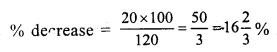Question 21.
Solution:
Rate of commission = 2% on first Rs. 200000
1 % on next Rs. 200000 and 0.5% on remaining price
Sale price of property = Rs.200000 + 200000 +140000 = Rs. 540000
Now commission earned by the
= Rs. 200000 x 2% + Rs. 200000 x 1% + 140000 x 0.5%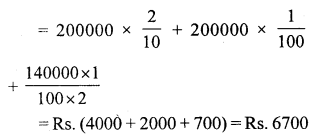Question 22.
Solution:
Let Akhil’s income = Rs. 100
Then income of Nikhil’s will be = Rs. 100 – 20 = Rs. 80
Amount which is more than that of Akhil’s = 100 – 80 = Rs. 20
% age = 20×10080 = 25%

Question 23.
Solution:
Let income of Mr Thomas = Rs. 100
then income of John = Rs. 100 + 20 = Rs. 120
Income of Mr Thomas is less than John = Rs. 120 – 100 = Rs. 20
% age = 20×100120
= 503 = 1623 %

Question 24.
Solution:
Present value of machine = Rs. 387000
Rate of depreciation = 10%
Let 1 year ago the value of machine was = x1 year ago, value of machine = Rs. 430000

Question 25.
Solution:
Present value of car = Rs. 450000
Rate of decreasing of value = 20%
Value after 2 yearsQuestion 26.
Solution:
Present population = 60000
Rate of increase = 10%
Increased population after 2 yearsQuestion 27.
Solution:
Let the price of sugar = Rs. 100
and consumption = 100 kg.
Increase price of 100 kg = Rs. 100 + 25 = Rs. 125
Now increased amount on 100 kg = Rs. 125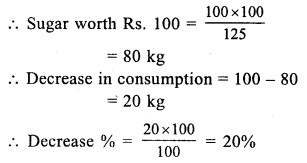All Chapter RS Aggarwal Solutions For Class 7 Maths

—————————————————————————–

All Subject NCERT Exemplar Problems Solutions For Class 7

All Subject NCERT Solutions For Class 7

*************************************************

I think you got complete solutions for this chapter. If You have any queries regarding this chapter, please comment on the below section our subject teacher will answer you. We tried our best to give complete solutions so you got good marks in your exam.

If these solutions have helped you, you can also share rsaggarwalsolutions.in to your friends.